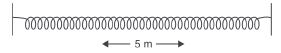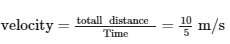Courses

# Sound, Science, Class 9 - Test

## 10 Questions MCQ Test Class 9 Science by VP Classes | Sound, Science, Class 9 - Test

Description
This mock test of Sound, Science, Class 9 - Test for Class 9 helps you for every Class 9 entrance exam. This contains 10 Multiple Choice Questions for Class 9 Sound, Science, Class 9 - Test (mcq) to study with solutions a complete question bank. The solved questions answers in this Sound, Science, Class 9 - Test quiz give you a good mix of easy questions and tough questions. Class 9 students definitely take this Sound, Science, Class 9 - Test exercise for a better result in the exam. You can find other Sound, Science, Class 9 - Test extra questions, long questions & short questions for Class 9 on EduRev as well by searching above.
QUESTION: 1

### A wave in slinky travelled to and fro in 5 sec the length of the slinky is 5 m. The velocity of wave isSolution:
As the wave moves to and fro so the total distance will be d=2x5=10 m

we have
time (t) = 5 shence velocity will be =2 m/s
QUESTION: 2

Solution:
QUESTION: 3

### Which characteristic is this? We can distinguish between sound having the same pitch and loudness.

Solution:
QUESTION: 4

Speed of sound depends upon

Solution:
QUESTION: 5

Speed (v), wavelength (λ) and frequency (ν) of sound are related as

Solution:

The time taken by the wave for one complete oscillation of the density or pressure of the medium is called the time period, T. The speed v, frequency ν, and wavelength λ, of sound are related by the equation, v = λν.

QUESTION: 6

To hear a distinct echo the time interval between the original sound and the reflected sound must be

Solution:
QUESTION: 7

Reverberation of sound is used in

Solution:
QUESTION: 8

Children under the age of 5 can hear upto

Solution:
QUESTION: 9

Dolphins, bats and porpoise use

Solution:
QUESTION: 10

The part of human ear that converts sound vibrations into electrical signals are

Solution: The eardrum converts sound into vibration and cochlea receive the vibrationnow the cochlea has to send the message to the brains. since brain can'tunderstand vibration cochlea converts soundvibration to the electrical signal which makes the brain easy to read.